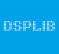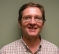## Gaussian noise generation using non-pseudo random number generatorsStarted by 5 years ago7 replieslatest reply 5 years ago421 views

Hello,

Is it possible to generate a digital noise signal with a Gaussian random distribution without using a pseudo random number generator?

For instance, the following article by Steve Smith:

...details using a pseudo random number generator to generate sample data which is added multiple times to generate a single sample of data (which adheres to a Gaussian random distribution).

In other words, ((pseudo random number sample 1) + (pseudo random number sample 2) + (pseudo random number sample 3) + ... + (pseudo random number sample N)) / N = Gaussian random distribution sample 1

However, if I wanted to generate a similar Gaussian random distribution sample of data, without using a pseudo random number generator (as it's possible that the pseudo random number generator being used has been backdoored / compromised). Could I do something similar using a "somewhat" random signal (like a recording of room tone / room ambience)?

In other words, rather than using a pseudo random number generator, I'd record some room tone / room ambience (for say 1 minute). And then add up the first 10 samples of data from my recording, divide that by 10, and arrive at my first Gaussian random distribution sample of data.

If this isn't possible, does anyone have any recommendations how this could be done using some non-pseudo random audio data?

Thank you,
Nelson

[ - ]Central random theorem is not good to Gaussian random generarion. Much better to use Box-Muller transformaton uniform pseudorandom. There are many uniform pseudorandom algorithms with different comlexety and period. Usung audio data for pseudorandom generation is bad idea. Audio signal is always correlated because analog filters and so on. I suggest to use L'Ecuyer algoritms for uniform random generation

[ - ]Instead of summing multiple independant noise sources, it is also possible to use an allpass filter to modify the distribution, call it a distribution filter. This allows noise sources like a 1-bit noise from a LFSR to be used, which has guaranteed whiteness. Have not yet figured out the ideal allpass coefficients/order for this though.

[ - ]As mentioned by dsplib, you can generate a Gaussianly distributed random sequence using a uniform pseudo-random generator using Box-Muller or related methods. If you want to avoid pseudo-random generators, you can use a source of truly random numbers  (e.g., voltage on a resistor or a Geiger counter or race conditions in digital circuits).

Unfortunately, most truly random sequences are not uniformly distributed, but can be made so by appropriate conditioning after which the aforementioned transformations can be used.

Y(J)S

[ - ]I think we must be very careful by using "true" random sources because theses things can be really random, but very difficult to make its realy white. Electrinic circuits, adc and so on can provide color noise instead white noise.

[ - ]One must be even more careful in using pseudorandom sources, as they are deterministic and not random at all. So, of course, it depends on the application.

If the random source is not flat enough for the application requirements, it is not difficult to design a whitening filter.

In any case, my remarks related to the original questioner's remark about not wanting to use non-pseudorandom data. I just re-read that remark and now see that the question was specifically about using random audio. I presume that this means sampling some noisy but stationary audio (e.g., air-conditioner noise). Here I agree that there will almost certainly be resonances that would need inverse filtering. Alternatively, once could use a pseudorandom integer generator to permute a buffer of such samples (choosing the buffer size long enough to enable eliminating short-term correlations).

Y(J)S

[ - ]In simulation I found that pseudo-random numbers worked pretty well.  The criteria is that they be un-correlated enough to look like random noise.  The trick is to shift them completely through so that one number looks unrelated to the next.  For instance, if there is a 32bit PRBS generator be sure it is shifted by 32 bits to the next sample and not just by one.  To get my waterfall curves to look right I had to make my own generator.  The usual "rand" function just didn't work well enough.

[ - ]Not sure why you want to avoid using a prng, nevertheless there are several options which may meet your requirements. As others have mentioned Box-Muller can transform uniformly distributed numbers to gaussian.

On linux systems you can use  /dev/random  or  /dev/urandom  for a "true" random source.

https://en.wikipedia.org/wiki//dev/random

https://en.wikipedia.org/wiki/Hardware_random_numb...

https://www.howtoforge.com/tutorial/how-to-install...

https://www.random.org/

The following is actually a prng but it's very simple and has very good properties.

http://www.pcg-random.org/

Lito